CBSE Sample Papers for Class 9 Science Paper 6 are part of CBSE Sample Papers for Class 9 Science. Here we have given CBSE Sample Papers for Class 9 Science Paper 6.

## CBSE Sample Papers for Class 9 Science Paper 6

 Board CBSE Class IX Subject Science Sample Paper Set Paper 6 Category CBSE Sample Papers

Students who are going to appear for CBSE Class 9 Examinations are advised to practice the CBSE sample papers given here which is designed as per the latest Syllabus and marking scheme as prescribed by the CBSE is given here. Paper 6 of Solved CBSE Sample Paper for Class 9 Science is given below with free PDF download solutions.

Time Allowed : 3 Hours
Max. Marks: 80

General Instructions

• The question paper comprises of two Sections, A and B. You are to attempt both the sections.
• All questions are compulsory. However an internal choice will be provided in two questions of 3 marks each and one question of five marks.
• All questions of Section A and all questions of Section B are to be attempted separately.
• Question numbers 1 to 2 in Section A are one-mark questions. These are to be answered in one word or in one sentence.
• Question numbers 3 to 5 in Section A are two-marks questions. These are to be answered in about 30 words each.
• Question numbers 6 to 15 in Section A are three-marks questions. These are to be answered in about 50 words each.
• Question numbers 16 to 21 in Section A are five-marks questions. These are to be answered in about 70 words each.
• Question numbers 22 to 27 in Section B are two-marks questions based on practical skills. These are to be answered in brief

Questions
SECTION-A

Question 1.
Convert the following into Celsius scale: 300 K and 573 K

Question 2.
An organism does not have a well defined nucleus and organelles. State its kingdom.

Question 3.
Name the compounds whose formulae are given below:

1. Ca(OH)2
2. KMnO4

Question 4.
Differentiate between infectious and non-infectious diseases.

Question 5.
Calculate the number of moles present in 60 gm of calcium.

Question 6.
State the law of constant proportion. Illustrate it with examples.

Question 7.
What is ultrasound? Give two uses of ultrasound.

Question 8.

1. Define atomic mass unit.
2. Write the chemical formula for potassium oxide and ammonium chloride.

Question 9.
A man is suffering from AIDS.

1. What is the cause of AIDS and give its full form.
2. Write two ways by which this disease gets transmitted.
3. State any one precaution for prevention of AIDS.

OR
Define the term “symbiosis”. Name a symbiotic life forms. Name the place where these life forms are found.

Question 10.
Distinguish between density and relative density of a substance. The relative density of silver is 10.8. If the density of water is 103 Kg/m3 find the density of silver.

Question 11.
Differentiate between radial symmetry and bilateral symmetry. Give one example of organism having this symmetry for each.

Question 12.

1. State Archimedes principle.
2. State the laws of floatation.
3. Why is it easier to swim in sea water than in river water.

OR
State Pascal’s law for pressure. Why tip of pin is sharp but the head is broad? How camels walk easily in desert soil.

Question 13.
Explain the Rutherford’s model of atom. Write his three observations and conclusions made by alpha particle splitting experiment.

Question 14.
Calculate the atomic mass of chlorine if it exists commonly in the form of two isotope. Cl mass number 35 – 75%, Cl mass number 37 – 25%. Name two isotopes which can cure diseases.

Question 15.
What are the different means by which infectious diseases are spread?

Question 16.
(a) Explain the various factors on which the rate of evaporation depends, giving example.
(b) Explain how the high compressibility of gases is useful.

Question 17.
How can you separate the following mixture:

1. Sand + iron
2. Cream from milk
3. Salt + water
4. Ammonium chloride + NaCl
5. Copper sulphate and water
6. Rice and dal (uncooked)
7. Gases from air
8. Petrol and diesel form crude oil
9. Drugs from blood
10. Acetone from water

Question 18.
(a) What is uniform circular motion?(b) An athlete runs on a circular track, whose radius is 50 m with a constant speed. It takes 50 seconds to reach point B from starting point A. Find
(i) the distance covered
(ii) the displacement
(iii) the speed.

Question 19.
(a) Describe on activity to obtain crystals of copper sulphate from its solution.
(b) Draw a flow chart to show the water purification system. Why is chlorination of water important?

Question 20.
What is a disease? How do you know if a person has got disease? List various causes for the disease.

Question 21.
Explain the physical, chemical and biological factors which lead to the formation of soil.
OR
Explain nitrogen-cycle in nature and define all the terms involved in it.

SECTION-B

Question 22.
Some raisins weighed 10 g before they were placed in water for four hours. The raisins were then removed, wiped and weighed again. Their weight was found to be 12.5 g. Calculate the percentage of water imbibed by them.

Question 23.
The given diagram shows the stage of mosquito,Give the name of this stage state two significant characteristics.

Question 24.
A student studied the different parts of seed. Lable the parts of seed studied by him.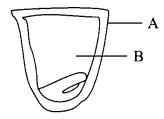Question 25.
Why does the temperature remain unchanged until the entire solid changes into liquid even if we are heating the solid?

Question 26.
A rectangular body has dimensions x and y (l and b). If its dimensions get doubled what will happen to its volume?

Question 27.
If in the first step the mixture consisting of sand, common salt and NH4Cl had been dissolved in water what would have been the difficulty in separation?

SECTION-A

300 K = 27 degree Celsius
573 K = 300 degree Celsius

Monera

1. Calcium Hydroxide
2. Potassium permanganate or Potassium manganite.

 Infectious Diseases Non-infectious Diseases It can be transferred from an infected person to a healthy person. It does not get transferred from an infected person to a healthy person. They are caused by pathogens and normally spread by some agents like air, water and contact, e.g. Flu. They are caused due to deficiency of minerals/vitamins or organ/tissue failure e.g. Kwashiorkor, Scurvy.

Given mass, m = 60 g
Molar mass, M=40 g mol-1
No. of moles, n= $$\frac { m }{ M }$$ = $$\frac { 60 }{ 40 }$$ = 1.5 mol

Law of constant proportion states that ‘a chemical compound is always made up of the same elements combined together in the fixed proportion by mass’.

Water is composed of hydrogen and oxygen combined together in the ratio 1:8 by weight.
Atomic mass of hydrogen = 2, Atomic mass of oxygen = 16, when they combine,
∴ Ratio = $$\frac { 2 }{ 16 }$$ = $$\frac { 1 }{ 8 }$$ = 1 : 8
In water (H2O) the ratio of hydrogen to oxygen is 1 : 8 in which 1 g of hydrogen and 8 g of oxygen is needed to form the compound (H2O).
Similarly in ammonia, the ratio of nitrogen to hydrogen is 14 : 3.

Ultrasound is the name given to the sound waves that have frequencies greater than 20000 Hz.
Uses of Ultrasound:

• Checking the growth of a foetus.
• Breaking down kidney stones and stones elsewhere in the body.
• Measuring the speed of blood flow in the body. (Any two)

1. The atomic mass unit is a unit used to express the atomic mass of atoms and molecular masses of compounds. One atomic mass unit is a mass unit exactly one twelfth of the mass of one atom of Carbon-12.
2. K2O and AlCl3

1. AIDS is caused by HIV virus, its full form Acquired Immuno Deficiency Syndrome.
2. It can be transmitted from an is infected person to another person by use of common syringe, through sexual contact etc.
3. Use of disposable syringes test before blood transfusion.

OR
Symbiosis is a relationship in which two organisms are associated and are mutually benefited. Lichen is an example of symbiotic life form. These life forms are found in pollution free environment, e.g. on the tree trunks, rocks, ground etc.

 Density Relative Density (i) It is defined as mass per unit volume. (i) It is defined as the ratio of density of the substance to density of water at 4°C. (ii) Its value is different in different systems of measurement. (ii) Its value is the same in all systems of measurement. (iii) Its units are g cm-3 and kg m-3. (iii) It has no units.

Relative density of silver =10.8
Density of water = 103 kg m-3
∴ RD = $$\frac { Densityofsilver }{ Densityofwater }$$
Density of silver = RD × Density of water
= 10.8 × 103 kg m-3

 Radial Symmetry Bilateral Symmerty (i) If the body of an animal is divided into two equal parts by cutting it through any plane passing through the centre of its body, then the body of the animal is said to have radial symmetry. (i) If the body of an animal is divided into left and right halves by only one plane, then the body of the animal is said to have bilateral symmetry. (ii) Animals of phylum coelenterate have radially symmetrical body. (ii) Animals of phylum helminthes and higher phyla have bilaterally symmetrical body. E.g. Hydra, Starfish E.g. Mammals and Birds

1. Archimedes Principle: a body totally or partially immersed in a fluid is subject to an upward force equal in magnitude to the weight of fluid it displaces.
• A freely floating body in a fluid displaces the weight of the fluid equivalent to its own weight.
• The centre of gravity of the freely floating body and the centre of gravity of the fluid are in the same vertical line,.
2. It is easier to swim in the sea water because it is denser than the river water and thus it can exerts more upthrust.

OR
Pascal’s law or tile principle of transmission of fluid-pressure states that “pressure exerted anywhere in a confined incompressible fluid is transmitted equally in all the directions throughout the fluid such that the pressure ratio (initial difference) remains same”.
The tip of the pin can be easily pierced into the cloth with less pressure exerted on the smaller area for larger impact.

Camel’s feet are broad and larger area of the feet reduces the force/pressure exerted by the body on the sand. But when we have to walk on the same sand, we sink because the pressure exerted by our body is not distributed, but it is directional.

Rutherford’s α-particle scattering experiment:
Fast moving α-particles were made to fall on a thin gold foil. These particles have + 2 charge and 4 u mass, and have considerable amount of energy. When they hit the gold foil the following observations are made: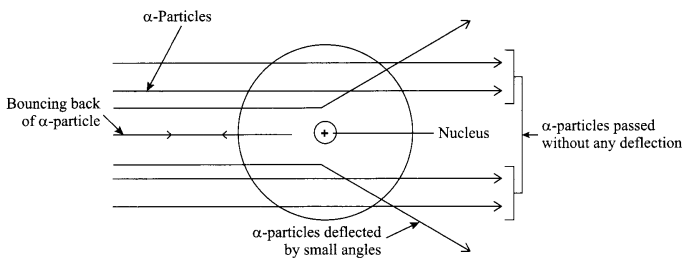• Most of the a-particles passed straight through the foil.
• Some of the a-particles were deflected by small angles through the foil.
• One out of every 20,000 particles were rebounded.

Conclusion:

1. Most of the space inside the foil is empty.
2. Positive charge of the atom occupies very less space.
3. Mass of the atom is concentrated in the centre of the atom with all positive charge concentrated in the small volume within the atom.

Average atomic mass = $$\frac { 75 }{ 100 }$$ × 35 + $$\frac { 25 }{ 100 }$$ × 37
=26.25 + 9.25 = 35.5 u
Cobalt-60 is used to cure cancer and Iodine-123 is used for thyroid treatment.

The different means by which infectious diseases spread are:

1. Through air: They are also called airborne diseases. Air carries bacteria, virus and the diseases that can be caused are common cold, influenza, tuberculosis etc.
2. Throujfn food and water: When one eats/drink contaminated food/water, that contains bacteria, virus, worms etc, it can cause diseases like cholera typhoid, hepatitis.
3. Through contact: Many diseases spread by contact of an infected person with the healthy person. Example, fungal infections, skin diseases, etc.
4. By sexual contact: Many diseases can be transmitted through sexual contact. Example, syphilis, AIDS.
5. By body fluids: Fluids like blood, semen, mother’s milk when infected can also cause diseases. Example, AIDS.
6. Vectors: The organisms that spreads diseases by carrying pathogens from one place to another are called vectors. Example, mosquitoes are vectors that carry pathogens like protozoa.

(a) The rate of evaporation depends on the following factors:

1. Surface area: If the surface area is increased the rate of evaporation also increases.
• To dry the clothes faster we spread them to dry.
• Tea in a saucer cools faster than in a cup.
2. Temperature: If the temperature is increased the rate of evaporation also increases. Due to increase in temperature the particles gain more kinetic energy and the phase changes from liquid to gaseous phase, e.g. water will evaporate faster in the presence of sun than in shade.
3. Humidity: It is the amount of water vapour present in air. The air can hold definite amount of water vapour, at a given temperature. If the amount of water vapour is high in the air then the rate of evaporation decreases.
On a hot and humid day, desert coolers are not effective as the air cannot hold anymore moisture to get the cooling effect.
4. Wind speed: With increase in wind speed, the rate of evaporation increases.
The particles of water vapour moves away with the wind, thereby decreasing the amount of water vapour in the surroundings.

(b) Gases have high compressibility. This property is used in the following situations:

1. LPG (liquefied petroleum gas) is a fuel which is made up of petroleum gas. On compressing this liquified petroleum gas, it forms liquid. Similarly CNG, i.e. compressed natural gas is used as a fuel in vehicles and at home.
2. Oxygen cylinders in the hospitals have compressed gas filled in it.

1. Magnetic separation
2. Centrifugation
3. Evaporation
4. Sublimation
5. Crystallisation
6. Hand picking
7. Distillation
8. Fractional distillation
9. Chromatography
10. Distillation

(a) When a body moves in a circular path with uniform speed, its motion is called uniform Circular motion.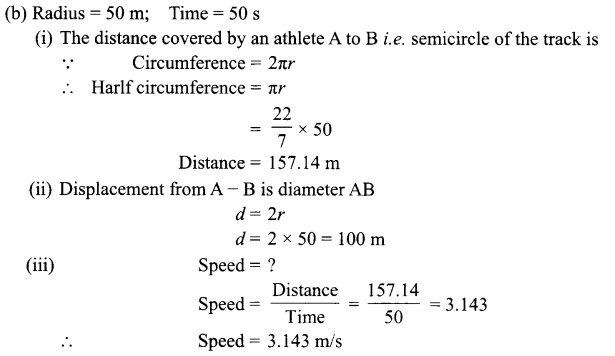(a) Activity demonstrating preparation of crystals of copper sulphate from its solution:

• Take a clean beaker (250 mL) and put the copper sulphate solution in it.
• Heat the solution in the beaker to 60 – 70° C with continuous stirring, on a wire gauze.
• Filter the solution and collect the filtrate in a china dish.
• Place the china dish over the wire gauze placed over a tripod stand and heat it gently (do not boil).
• As the solution gets heated, stir it using a glass rod. This helps in uniform evaporation and also prevents the formation of solid crust.
• When the solution is reduced to one-half of is volume, take out a drop of the concentrated solution from one end of the glass rod and cool it by blowing air. Formation of thin crust indicates that crystallisation point is achieved.
• Turn off the burner, cover the dish with a watch glass, and keep it undisturbed. As the solution cools down, crystals will separate out. Slow cooling ensures better crystallisation.
• Decant the mother liquor and wash the crystals with a thin stream of cold water and dry the crystals by pressing them gently between the sheets of filer paper.
• Blue coloured crystals of copper sulphate (CuSO4 . 5H2O) are formed).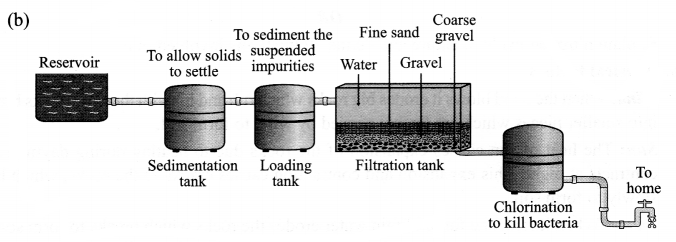Chlorination of water helps to check the growth of disease causing microbes, e.g. bateria, protozoa, etc. and it thus helps in preventing waterborne diseases. Thus, chlorination of water is important.

Disease is a condition in which an individual suffers from one or more type of illness/ is not in the state of being called as healthy.
Common methods of transmission of diseases: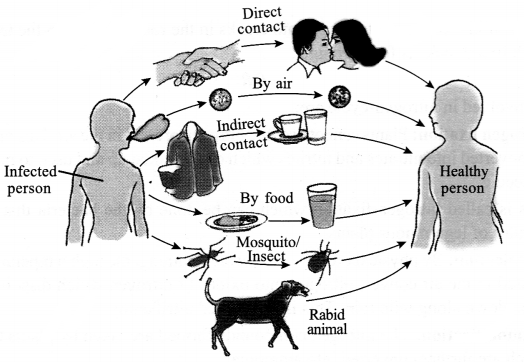The common methods of transmission of diseases are:

• By air – cough, cold, tuberculosis
• By food and water – typhoid, jaundice
• By mosquito bite – malaria
• By rabid animal – rabies
• By direct contact – skin infection, small pox, AIDS
• By indirect contact – typhoid, chicken-pox

Physical factors:

• Wind: When the wind blows it erodes big rocks which rub and hit with the other rocks breaking into smaller pieces which are further carried by wind to form soil.
• Sun: The heat of sun causes expansion of the rocks due to heating during daytime and it contracts at night. This expansion and contraction causes cracks in the rocks, which breaks slowly to form soil.
• Water: When it rains the force of rain water erodes the rocks which breaks to form soil.
At times this water enters the cracks present in the rocks and freezes when temperature falls which breaks the rocks to forms soil.

Chemical factors:

• Water: Water when reacts with carbonates and phosphates present in the rocks, it form soluble compounds which make the rock hollow from inside.
• Biological factor: Living organisms also help in the formation of soil. The lichen that grows on moist surface of rocks releases certain substances that causes the rock surface to powder down and form thin layer of soil. Other small plant like moss are able to grow on this surface now.
The roots of big trees sometimes go into cracks in the rocks and when the tree grows bigger the crack in the rocks is forced bigger.

OR
Terms involved in nitrogen-cycle are:

1. Nitrogen fixation: Plants cannot use free nitrogen present in the air. This nitrogen molecule is converted into nitrates and nitrites which can be taken up and used to make the required molecule.
This is called nitrogen fixation which can be done by the bacteria that live in the root nodules of leguminous plants.
2. Nitrification: By physical process, i.e. during lightning, the high temperature and pressure created in the air convert nitrogen into oxides of nitrogen which dissolves in water and came down along with rain. This is also called nitrification.
3. Ammonification: The nitrogen compounds formed are taken by plants to form proteins which are further converted into ammonia.
4. Denitrification: The nitrates and nitrites of nitrogen are acted upon by other group of microbes e.g. Pseudomonas bacteria, which convert these compounds into free nitrogen gas.

Nitrogen cycle:

1. Free nitrogen from atmosphere is converted into nitrates by bacteria or by lightning.
2. Nitrates mix with soil, is absorbed by the plants to make proteins.
3. The proteins in plants and animals are converted into amino acids and ammonia.
4. Ammonia is converted into nitrates and then these nitrates and nitrites present in soil is acted upon by other group of bacteria called denitrifying bacteria. This process is called denitrification.
5. Nitrates are converted into free nitrogen and is released back to the atmosphere.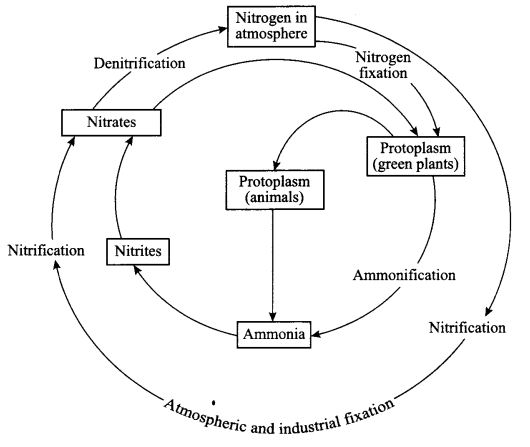SECTION-B

Weight of raisins (x) = 10 g
Weight of swollen raisins (y) = 12.5 g
The percentage of water absorbed by the raisins = $$\frac { y-x }{ x }$$ × 100
= $$\frac { 12.5 – 10 }{ 10 }$$ × 100 = 25%

This stage is called Tumblers.
The significant characteristics are it floats on water and takes oxygen through he tube named trumpets. This is the hibernating stage.

• A – seed-coat
• B – cotyledon

The temperature remains unchanged until the entire solid changes into liquid even if we are heating the solid; it is because the heat is used up by the molecules in overcoming the forces of attraction between the parcticles of the solid.